Latest Teaching jobs   »   CTET 2014 Maths Pedagogy Questions: Free...

# CTET 2014 Maths Pedagogy Questions: Free PDF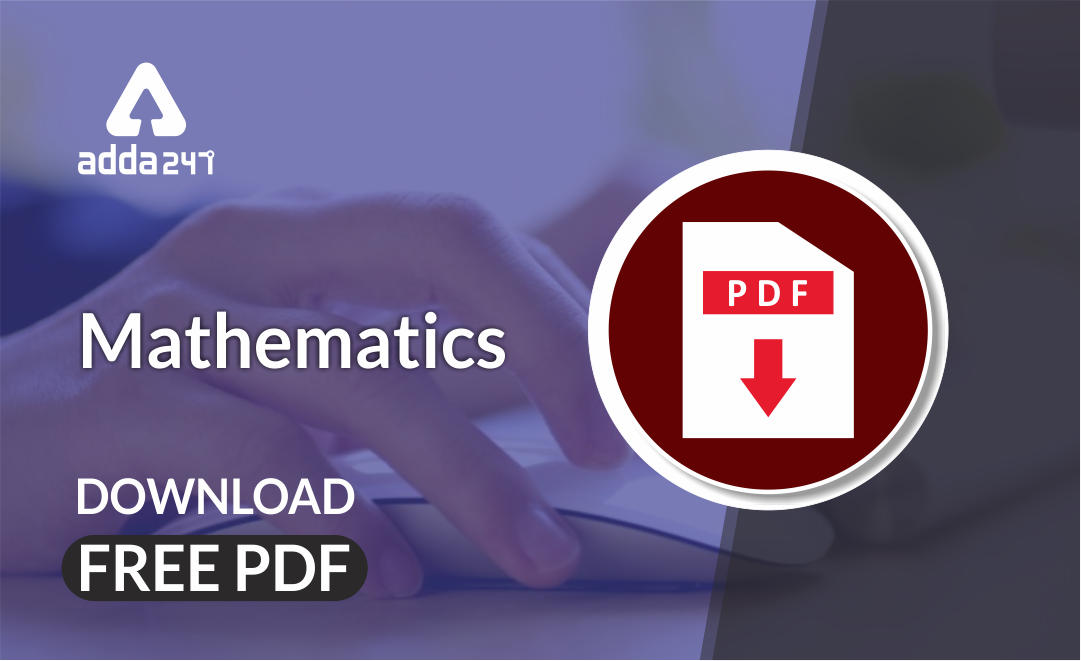Mathematics is an equally important section for CTET, MPTET, KVS & DSSSB Exams and has even more abundant importance in some other exams conducted by central or state govt.

To let you make the most of Mathematics section, we are providing important previous year Maths Pedagogy Questions.  We wish you all the best of luck to come over the fear of the Mathematics section.

Mathematics Study Notes For All Teaching Exams

## CTET 2014(Paper 2) Maths Pedagogy Questions

Q1. A teacher conducted, a debate in the class on the following topic: “Zero is the most significant number.” She encouraged every child to express his / her view on the topic. The teacher is

(a) making her classroom more communicative and reflective

(b) passing her time as students are not in mood of studying

(c) using her Mathematics class as life – skill class to develop value of argument among the

children

(d) inducing problem – solving skill among the children

Q2. Under the topic, “Use of exponents to express small numbers in standard form”, the following facts are stated:

1. The speed of light is 300000000 m/sec.
2. The height of the Mount Everest is 8848 m.
3. The diameter of a wire on a computer chip is 0.000003 m.
4. The size of a plant cell is 0.00001275 m.

The above examples are used to express each stated number in standard form. Use of such examples

(a) shows the accuracy aspect of numbers

(b) reflects the inter – disciplinary approach

(c) is made in class to grab the attention of students more interested in science

(d) helps the teacher to identify the bright students

Q3. According to Jean Piaget’s theory

(a) introduction of linear equation and learning techniques of solving it are assimilation, and extending the concept to pair of linear equations may raise problem of accommodation

(b) introduction of pair of linear equations and techniques of solving it are assimilation, and connecting it to basics of linear equation is accommodation

(c) exposure to linear equation, techniques of solving it and pair of linear equations are

assimilation

(d) learning the concept of linear equation, pair of linear equations and quadratic equations is problem of accommodation

Q4. ‘Maths lab activities’ can be used for

(a) formative assessment only

(b) summative assessment only

(c) both formative as well as summative assessment

(d) selecting students for National Mathematics Olympiad

Q5. As per the NCF, 2005

(a) narrow aim of teaching Mathematics at school is to teach number system and higher aim is to teach algebra

(b) narrow aim of teaching Mathematics at school is to teach calculation and higher aim is to teach measurements

(c) narrow aim of teaching Mathematics at school is to develop numeracy – related skill and

higher aim is to develop problem – solving skill

(d) narrow aim of teaching Mathematics at school is to teach arithmetic and higher aim is to teach algebra

Q6. According to Bloom’s revised taxonomy, the cognitive objective that can be achieved through the following task “Prepare a PowerPoint presentation on contribution of Indian mathematicians.” is

(a) remembering

(b) understanding

(c) analyzing

(d) creating

Q7. Read the approaches used by the two teachers to teach solving of linear equation, say 2x – 6 = 10:

 Teacher A Teacher B Steps Steps A. Take 6 on other side 1. Equation always maintains equality. So same operation with same number can be performed on both sides to maintain equality B. Change the sign of 6 and add to 10 2. Hence, 2x – 6 + 6 = 10 + 6 or 2 = 16 C. Get 2x = 16 3.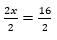or x = 8 D. Take 2 on the other side and divide E. Get x = 8

It can be observed that

(a) teacher A emphasizes on instrumental understanding while teacher B emphasizes on

relational understanding

(b) teacher A emphasizes on relational understanding while teacher B emphasizes on

instrumental understanding

(c) teacher A focuses on conceptual knowledge while teacher B focuses on procedural

knowledge

(d) teachers A and B focus on instrumental understanding

Q8. Class VI students were given the following layout of a house.

The students were asked to find out the –

(A) perimeter and area of each room;

(B) total perimeter and total area of the house.

The above activity can be used by teacher as formative assessment task because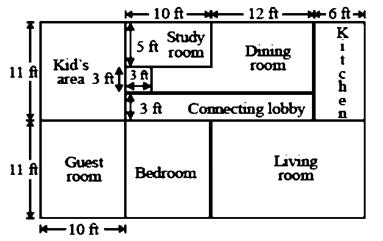(a) it will be easy for teacher to engage all the students for 40 minutes period

(b) the students’ responses will help teacher to diagnose their understanding, regarding finding

(c) dimensions, calculations, knowledge of formulae for perimeter and area, etc. the students will find the task interesting and will not disturb each other

(d) it will remove the phobia of Maths examination

Q9. Students’ ability to apply the concept of square roots in real life situation can be assessed through the following problem

(a) Find the smallest square number that is divisible by each of the numbers 4, 9 and 10.

(b) Calculate the square root of 25600.

(c) Find the smallest number that may be subtracted from 5607 to get a perfect square.

(d) 2025 plants are to be planted in a garden in such a way that each row contains as many plants as the number of rows. Find the number of rows and number of plants in each row.

Q10. A student writes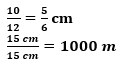This student

(a) always commits clerical error of writing unit with ratio

(b) has clear concept of units and their conversion

(c) can reduce fractions to lowest term correctly and can write the units properly

(d) has concept of units, conversion of units, fractions but missed the concept that ratio does not have units

Q11. “It is more useful to know how to mathematise than to know a lot of Mathematics”. This statement is given by

(a) David Wheeler

(b) George Polya

(c) Van Hiele

(d) Vygotsky

Q12. As per NCF 2005, one main goal of Mathematics education in schools is to

(a) develop numeracy skills

(b) enhance problem solving skills

(c) nurture analytical ability

(d) mathematise the child’s thought process

Q13. As per NCF 2005, Mathematics curriculum is ambitious, coherent and teaches important Mathematics. Here ‘ambitious’ refers to

(a) seek narrow aims Mathematics in school

(b) seek higher aims of teaching Mathematics in school

(c) teach more than one way of solving problems of Mathematics

(d) teach variety of Mathematics like arithmetic, algebra, geometry and data handling

Q14. Anil is able to answer all questions orally, but commits mistakes while writing the solutions of problems. The best remedial strategy to remove errors in his writing is

(a) giving him an assignment of 10 problems every day

(b) calling him out to solve a problem on the blackboard

(c) providing him with a worksheet with partially solved problems to complete the missing gaps

(d) giving him practice test after school hours, continuously for one month

Q15. A teacher asked the students to collect leaves and to identify symmetry patterns. This task reflects the teacher’s efforts to

(a) relate real life experience with mathematical concepts

(c) enhance creativity amongst students

(d) improve mathematical communication

Q16. The twin premises to fix the place of Mathematics teaching in our school curriculum are

(a) “how to engage the mind of every student” and “how to strengthen the student’s resources.”

(b) “how to improve the reasoning ability of every student” and “how to enhance his spatial

ability.”

(c) “how to raise the performance of every student in Mathematics” and “how to prepare

(d) “how to make the Mathematics class more activity oriented” and “how to enhance the

procedural skills and understanding of algorithms in every student.”

Q17. A teacher asked the students to “find the number of possible pentominoes using 5 squares and then further explore the number of possible hexominoes and so on.” These types of activities help the child to

(a) improve the observation skills

(b) identify relation between number pattern and shapes

(c) improve spatial ability

(d) improve analytical ability

Q18. As per the vision statement of NCF 2005, School Mathematics does not take place in a

situation, where children

(a) learn to enjoy Mathematics

(b) see Mathematics as a part of their daily life experience

(c) pose and solve meaningful problems

(d) memorise formulae and algorithms

Q19. With the help of ‘GeoGebra’ software, students can learn all concepts of geometry through

(a) exploratory approach

(b) inquiry – based approach

(c) project – based approach

(d) lecture – based approach

Q20. One of the major reasons for student’s failure in Mathematics at school level is that our assessment process

(a) emphasizes on testing of procedural knowledge than mathematisation of abilities

(b) is gender biased and asks problems relevant to boys’ interests

(c) is more subjective in nature and less or no objective type of questions are included

(d) gives more weightage to formative assessment than summative assessment

Mathematics Quiz For CTET Exam : Attempt Daily Quizzes

Solutions

S1. Ans.(a)

Sol. Zero is the most significant number’. Teacher makes her classroom more communicative and reflective

S2. Ans.(b)

Sol. Inter – disciplinary approach to teaching requires planning that looks at the foundational objectives of a number of curriculum areas. Connecting curriculum in the interdisciplinary approach is an effective way to help teachers deal with knowledge that grows at exponential proportions. A unit of study that uses the interdisciplinary approach enables teachers to teach the whole student and make links between discipline.

S3. Ans.(a)

Sol. Assimilation and accommodation are two key concepts of Jean Piaget’s theory. The process of taking in new information into our previously existing schemas is known as assimilation. Another part of adaptation involves changing or altering our existing schemas in light of new information, a process known as accommodation.

S4. Ans.(a)

Sol. Formative assessment is a range of formal and informal assessment procedure employed by teachers during the learning process in older to modify teaching and learning activities and to improve student attainment

S5. Ans.(c)

Sol. As per the NCF, 2005 problem – solving is the central focus on the mathematics curriculum. It is a process permeating the entire mathematics programme.

S6. Ans.(d)

Sol. Bloom’s taxonomy refers to a classification of the different objectives that educators set for students. The given task comes in creating category. Creation is to compile information together in a different way by combining elements in a new pattern or proposing alternative solutions.

S7. Ans.(a)

Sol. Instrumental understanding is having a mathematical rule and being able to use and manipulate it. Relational understanding is having a mathematical rule, knowing how to use it, and knowing why it works.

S8. Ans.(b)

Sol. Formative assessment including diagnostic testing is a range of formal and informal assessment procedures conducted by teachers during the learning process in order to modify teaching and learning activities to improve student attainment.

S9. Ans.(d)

Sol. 2025 plants are to be planted in a garden in such a way that each row contains as many plants as the number of rows. Find the number of rows and number of plants in each row.

S10. Ans.(d)

Sol. This has concept of units, conversion of units, fractions but missed the concept that ratio does not have units.

S11. Ans.(a)

Sol. David John Wheeler was a computer scientist. Wheeler’s contributions to the field included work on the EDSAC and the Burrows Wheeler transform

S12. Ans.(d)

Sol. The main goal of mathematics education in schools is the mathematisation of the child’s thinking, Clarity of thought and pursuing assumptions to logical conclusions is central to the mathematical enterprise. There are many ways to thinking and the kind of thinking one learns in mathematics is an ability to handle abstractions, and an approach to problem solving.

S13. Ans.(b)

Sol. As per NCF 2005, Mathematics curriculum is ambitious, coherent and teaches important Mathematics. Here ‘ambitious’ refers to seek higher aims of teaching Mathematics in school.

S14. Ans.(c)

Sol. Remedial instruction is the process of providing help to students who are experiencing difficulties so they can understand and master the concept with which they are struggling.

S15. Ans.(a)

Sol. A teacher asked the students to collect leaves and to identify symmetry patterns. This task reflects the teacher’s efforts to relate real life experience with mathematical concepts.

S16. Ans.(a)

Sol. “how to engage the mind of every student” and “how to strengthen the student’s resources.”

S17. Ans.(b)

Sol. These types of activities help the child to identify relation between number pattern and shapes.

S18. Ans.(d)

Sol. School Mathematics does not take place in a situation where children memories formulae and algorithms.

S19. Ans.(a)

Sol. GeoGebra is an interactive geometry, algebra, statistics and calculus application, intended for learning and teaching mathematics and science from primary school to university level.

S20. Ans.(a)

Sol. One of the major reasons for students’ failure in Mathematics at school level is that our assessment process emphasizes on testing of procedural knowledge than mathematisation of abilities.

•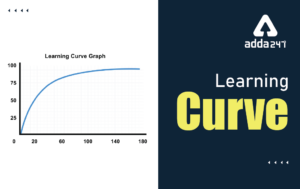Learning Curve - What is Learning Curve?...
•रस - परिभाषा, भे�...
•पठन कौशल- परिभा�...
•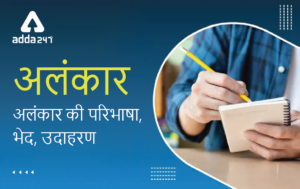अलंकार - परिभाष�...
•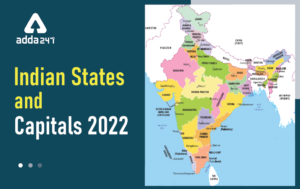Indian States and Capitals 2022: 28 Stat...
•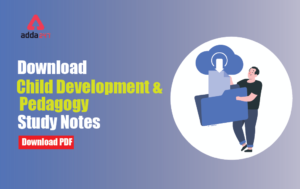New Education Policy(NEP) PDF - नई �...